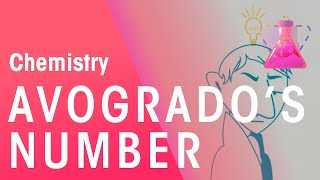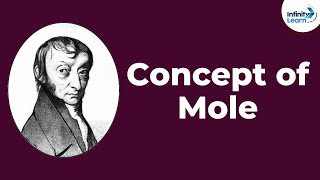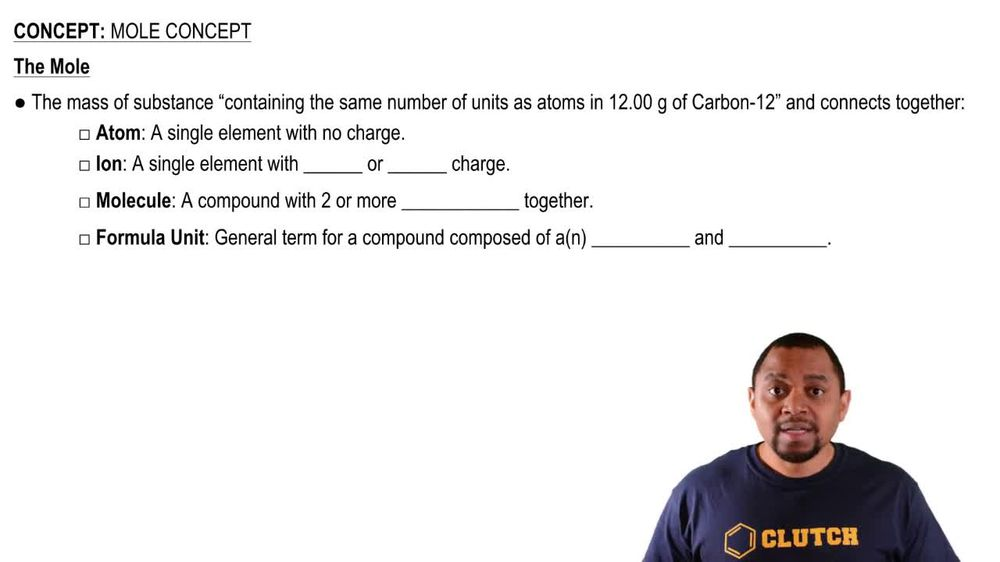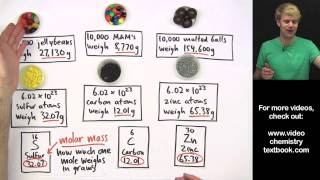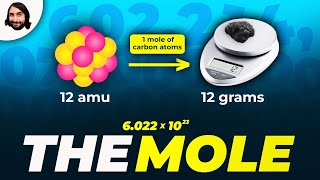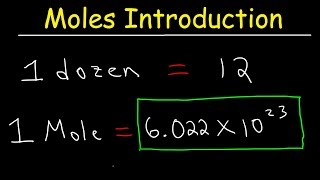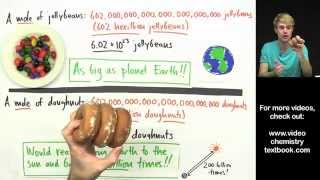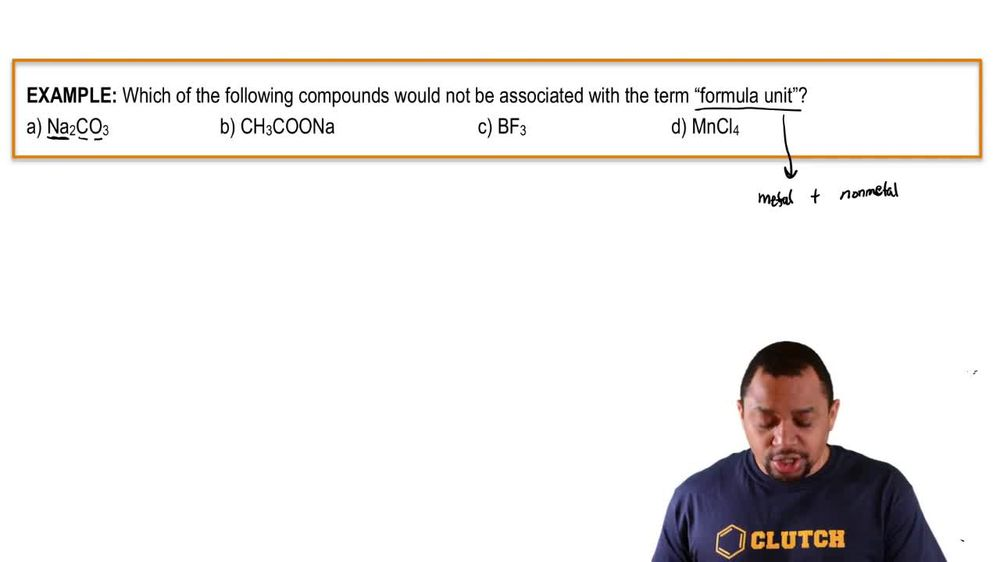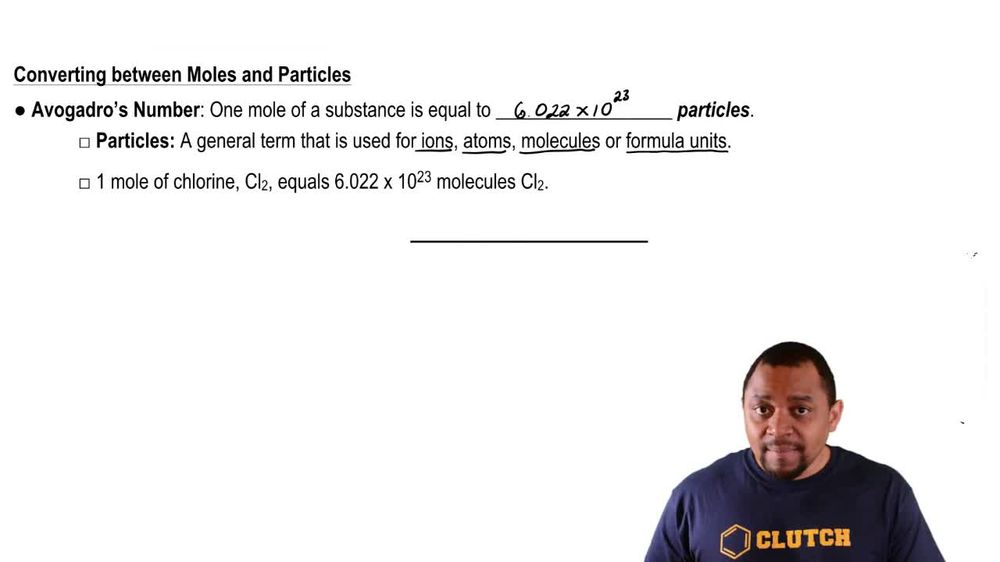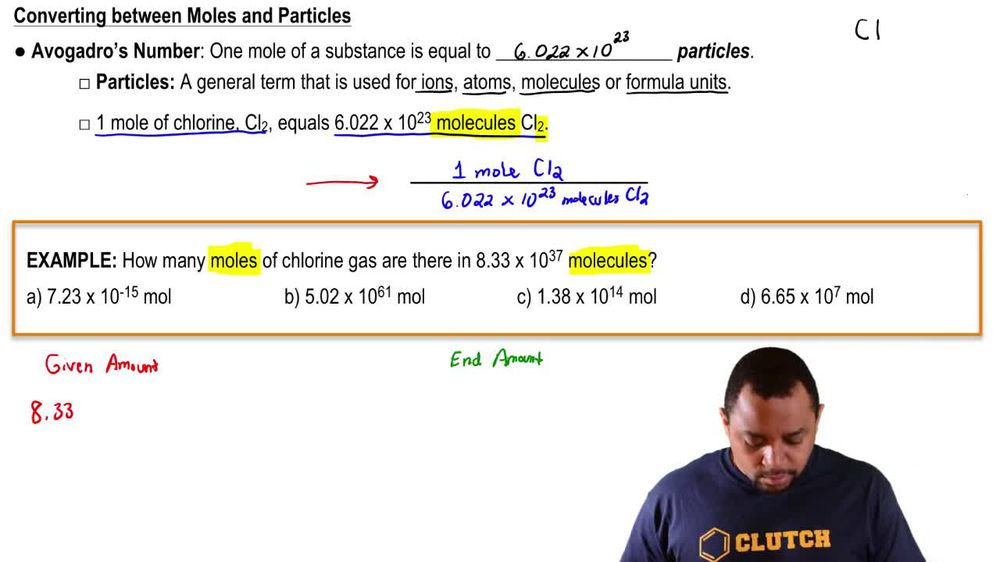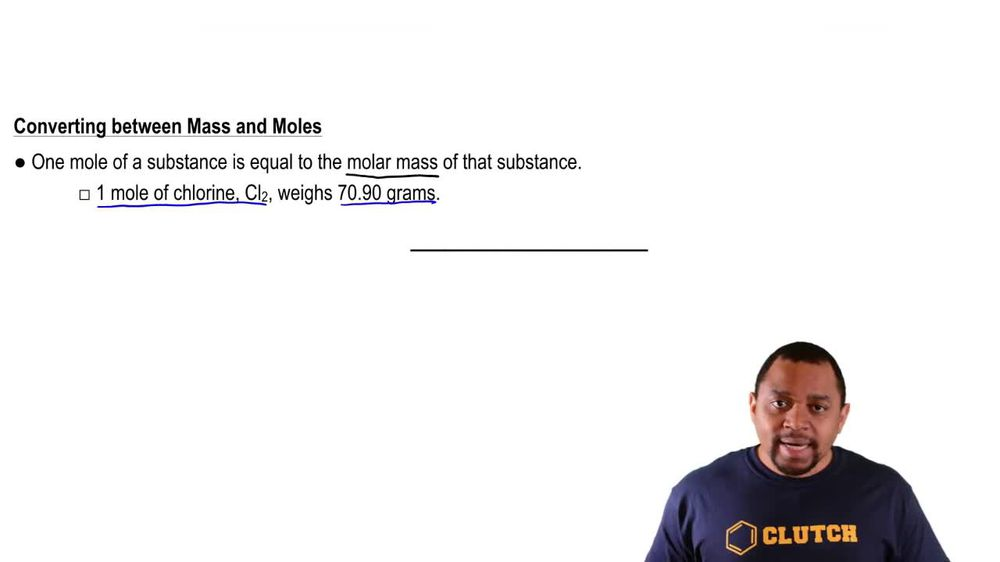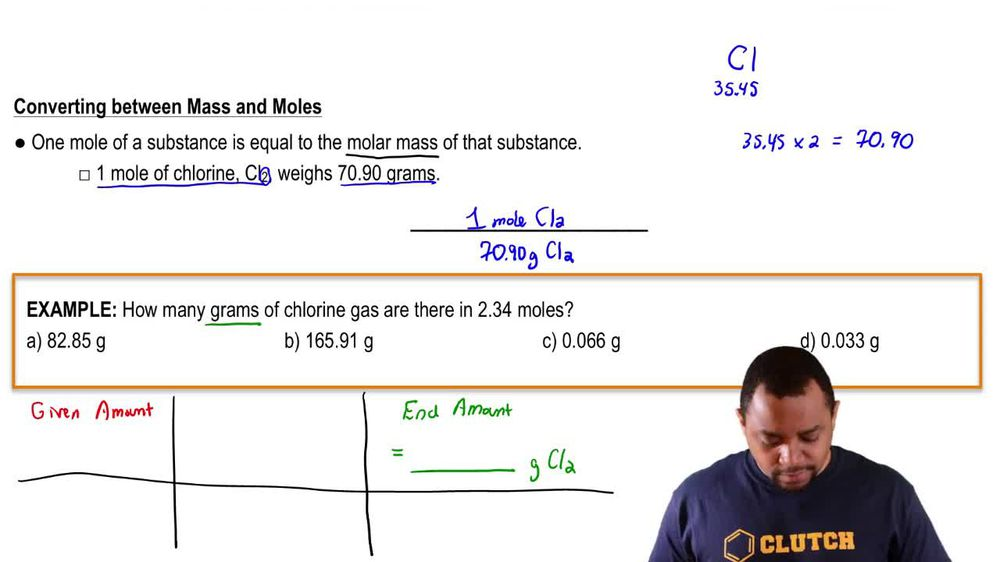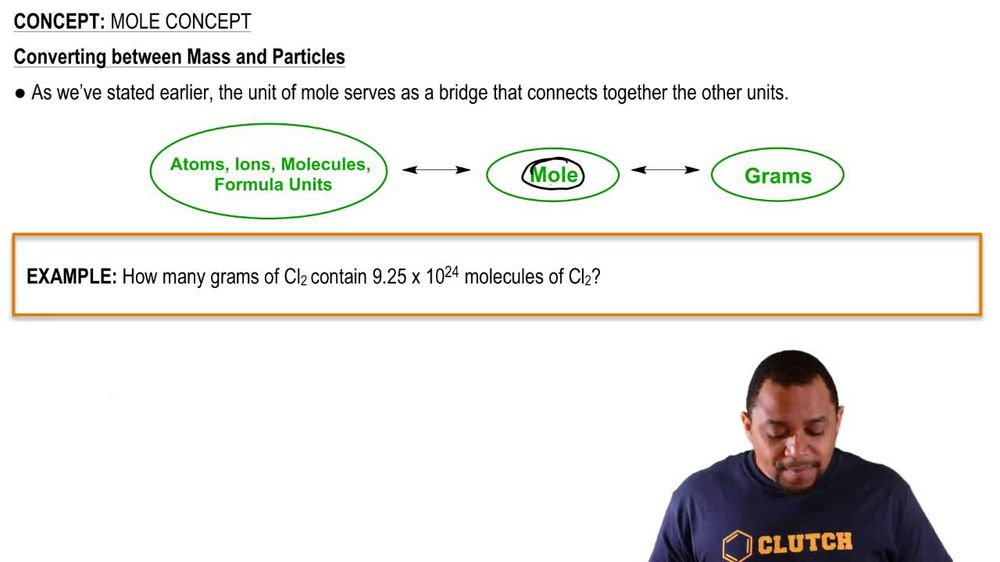Start typing, then use the up and down arrows to select an option from the list.
1. 2. Atoms & Elements2. Mole Concept
Problem

# Ammonium nitrate, a potential ingredient of terrorist bombs, can be made nonexplosive by addition of diammo-nium hydrogen phosphate, (NH4)2HPO4. Analysis of such a NH4NO3 - (NH4)2HPO4 mixture showed the mass percent of nitrogen to be 30.43%. What is the mass ratio of the two components in the mixture?

Relevant Solution5m
Play a video:
Hello everyone today. We have the following question. The active ingredients of a specific brand of fertilizers are mono, ammonium phosphate or M. A. P. And di ammonium phosphate or D A. P. Analysis of a mono or a map. Dap fertilizer revealed that it contained at 25.85% phosphorus by mass, calculate the mass ratio of map to DAp. So first we want to realize, we want to make note of this fertilizer having 25.85% phosphorus by mass. And so if we assume that we have 100 g of fertilizer, This would contain 25.85 g of phosphorus. The second thing we want to do is we want to determine our variables. So if we let X equal the mass of M A P. Or mono ammonium phosphate to find depth, we simply Would take g -X. And so third we want to determine the molar masses of our map and the molar mass of our depth. And so for map. Mono, mono, ammonium phosphate. That's going to be 115.06 g per mole. According to the periodic table and for adapt or die ammonium phosphate, that's going to be 132.06 g per mole. And so we can also assume that in each compound we will have one mole of phosphorus per one formula unit. And so what is that going to look like? Ultimately we're going to want to find our mass of map. And so we're going to take our 25. g of phosphorus. We're going to equal that to a very long and complicated equation. And so what is that gonna look like? Well we have our X. Which is representative of M a P. Or map. We're going to divide that by our molar mass of the map, which is 1 15.06 grams from all. We're going to close that off. And then we are also going to add the mass of D A P, which would be 100 minus X over the molar mass of D ap di ammonium phosphate, Which as you said before was 132.06 g per mole. We're going to close that off with brackets and then we're going to multiply by our conversion factor that one mole of phosphorus is going to equal one mole of our overall compound. Lastly We are going to multiply that by our 30.97 g of phosphorus equaling one mole of phosphorus. And that is essentially our molar mass of phosphorus. And so through all of these calculations, we are going to arise at the mass of our M A P or mono ammonium phosphate, which is going to equal 69. grams. But this is not our final answer, if we go to the top right here for set five to find the mass of Dap. So the mass of D A P or di ammonium phosphate. We're going to take that 100 minus x. Which is the mass of our M a p. Or 69.24 g. And that is going to give us 30. g for our DAP. And so the last step or step six is going to find is going to be to find the mass ratio of M A P. Mono ammonium phosphate two D ap di ammonium phosphate. And so that's simply going to be taking their masses and dividing them. So the mass of M A p is 69.24 g and are massive. D A p is 30.76 g. That gives us 2.25, which we can estimate to be just around two. And so ultimately, our mass ratio of our mono ammonium phosphate to our di ammonium phosphate is going to be 2 to 1. So for every two M ap we have one D a P. And with that we have answered this question. Ultimately, I hope this helped. And until next time